You are currently offline. Some features of the site may not work correctly.

# Monochromatic triangle

In graph theory and theoretical computer science, the monochromatic triangle problem is an algorithmic problem on graphs,in which the goal is to… Expand
Wikipedia

## Papers overview

Semantic Scholar uses AI to extract papers important to this topic.
2019
2019
• Graphs and Combinatorics
• 2019
• Corpus ID: 203031156
Let S be a set of N points on the plane in general position, colored arbitrarily with c colors ($$c\in {\mathbb {N}}$$). A subset… Expand
Is this relevant?
2017
2017
• IEEE 58th Annual Symposium on Foundations of…
• 2017
• Corpus ID: 11307924
Ramsey theory assures us that in any graph there is a clique or independent set of a certain size, roughly logarithmic in the… Expand
•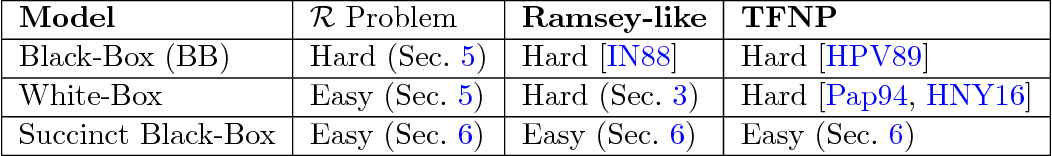•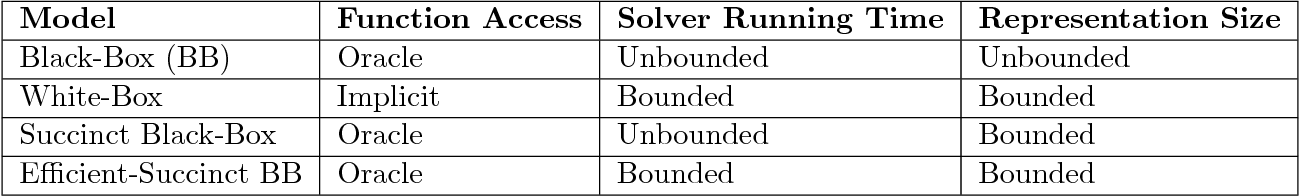Is this relevant?
2014
2014
• Discret. Appl. Math.
• 2014
• Corpus ID: 35701231
We study a combinatorial game called Bichromatic Triangle Game, defined as follows. Two players R and B construct a triangulation… Expand
•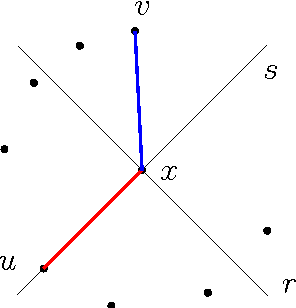•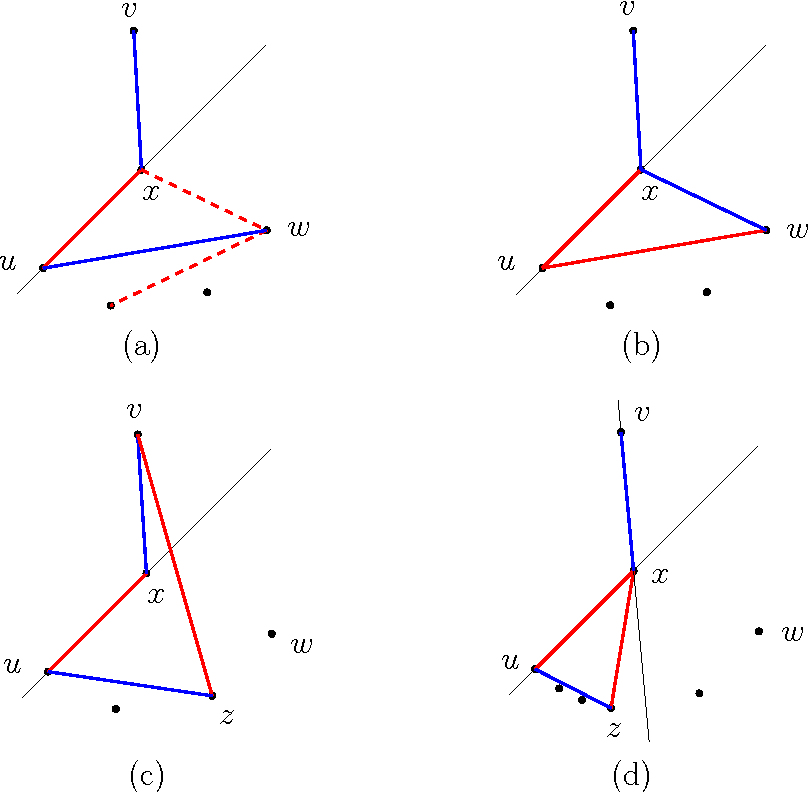•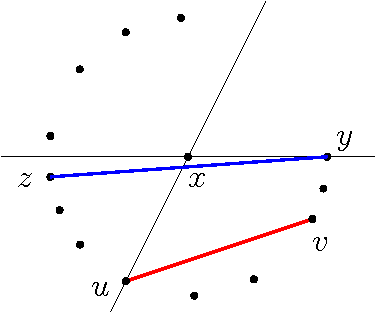•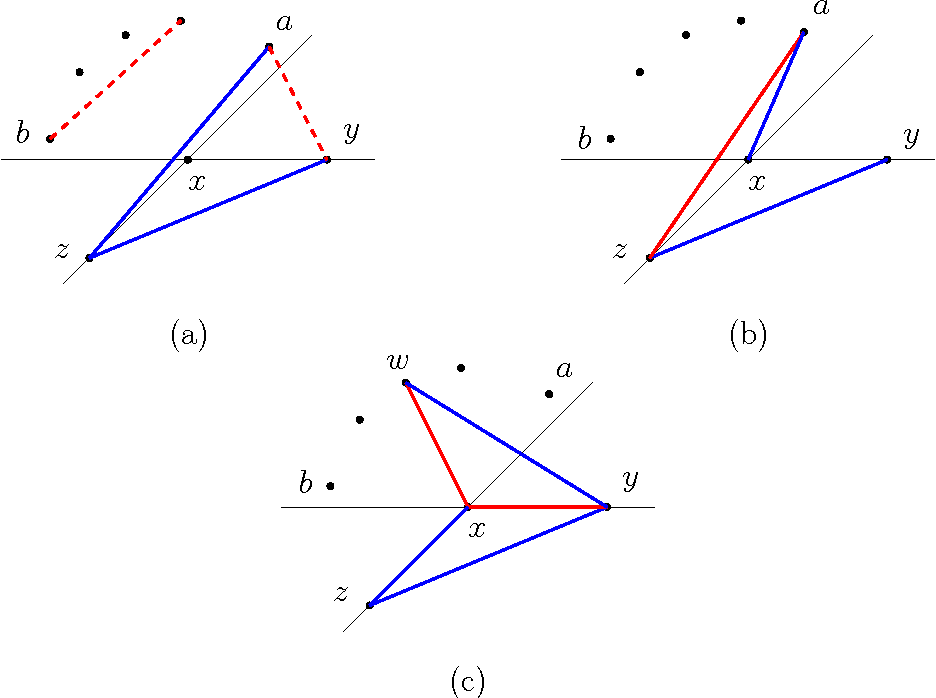•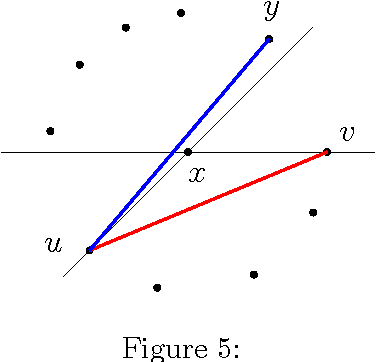Is this relevant?
2013
2013
• Electron. Notes Discret. Math.
• 2013
• Corpus ID: 33530134
For positive integers c, s⩾1, let M3(c,s) be the smallest integer such that any set of at least M3(c,s) points in the plane, no… Expand
•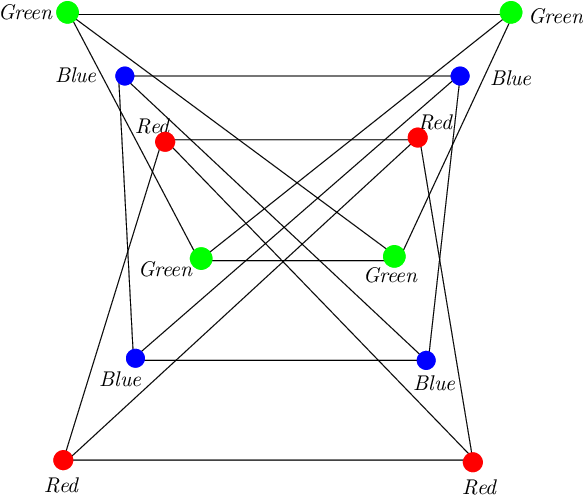•Is this relevant?
2008
2008
We prove that, for every list-assignment of two colors to every vertex of any planar graph, there is a list-coloring such that… Expand
Is this relevant?
2008
2008
• Discret. Math.
• 2008
• Corpus ID: 5664827
We give a very short proof of the following result of Graham from 1980: For any finite coloring of R^d, d>=2, and for any @a>0… Expand
Is this relevant?
2006
2006
• Memoirs of the American Mathematical Society
• 2006
• Corpus ID: 9143933
Let R be the set of all finite graphs G with the Ramsey property that every coloring of the edges of G by two colors yields a… Expand
•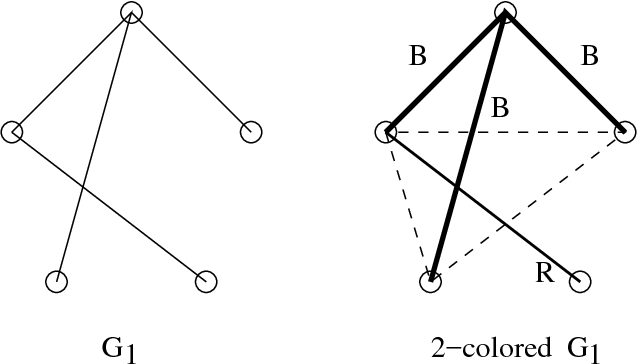•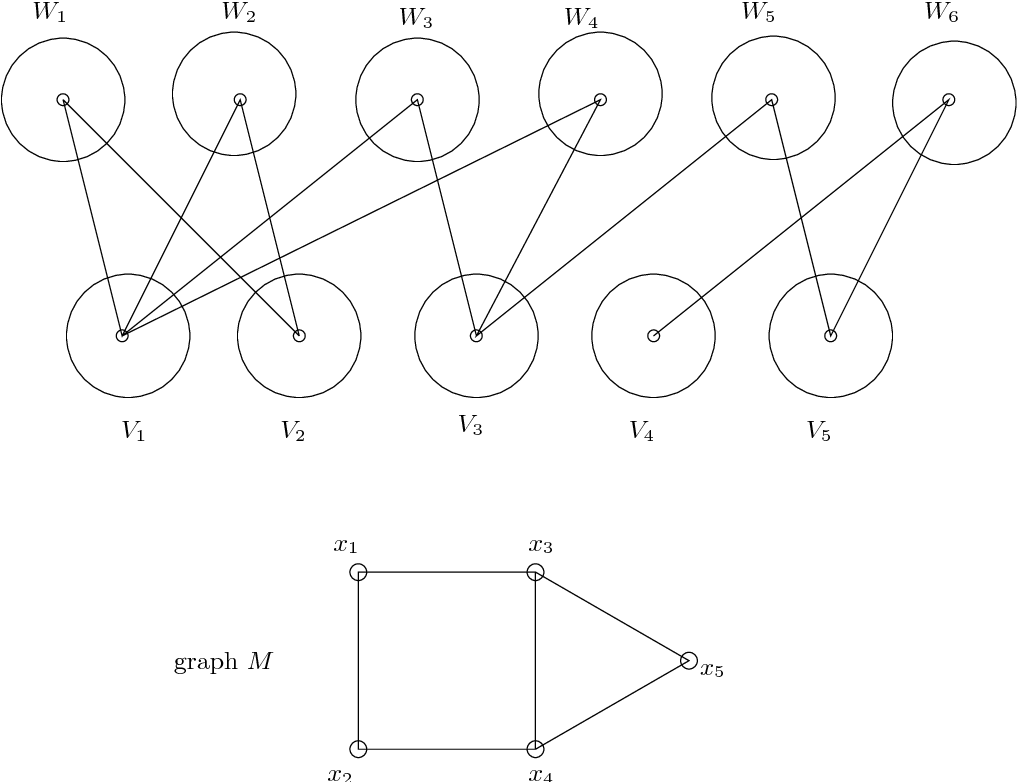•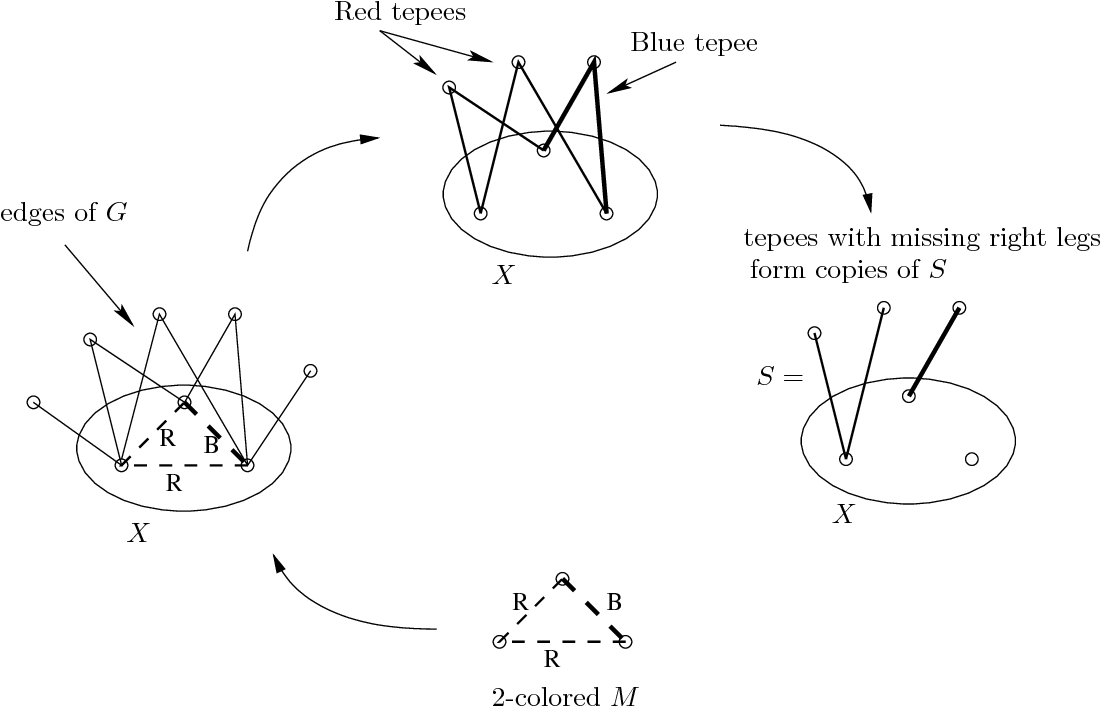•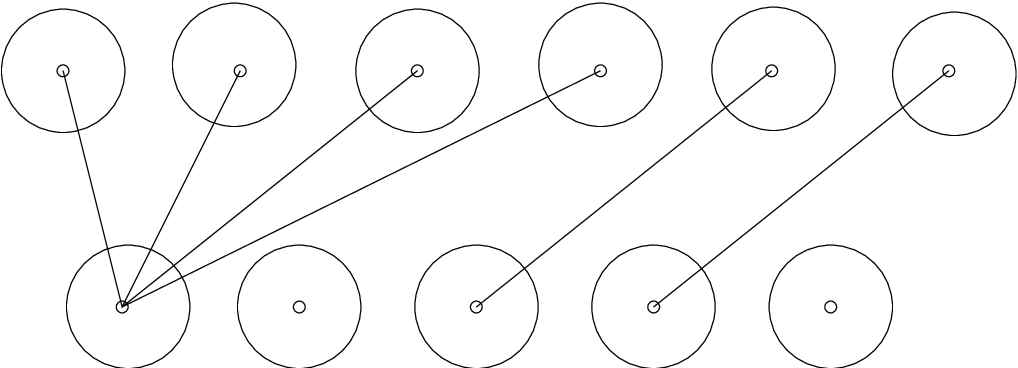•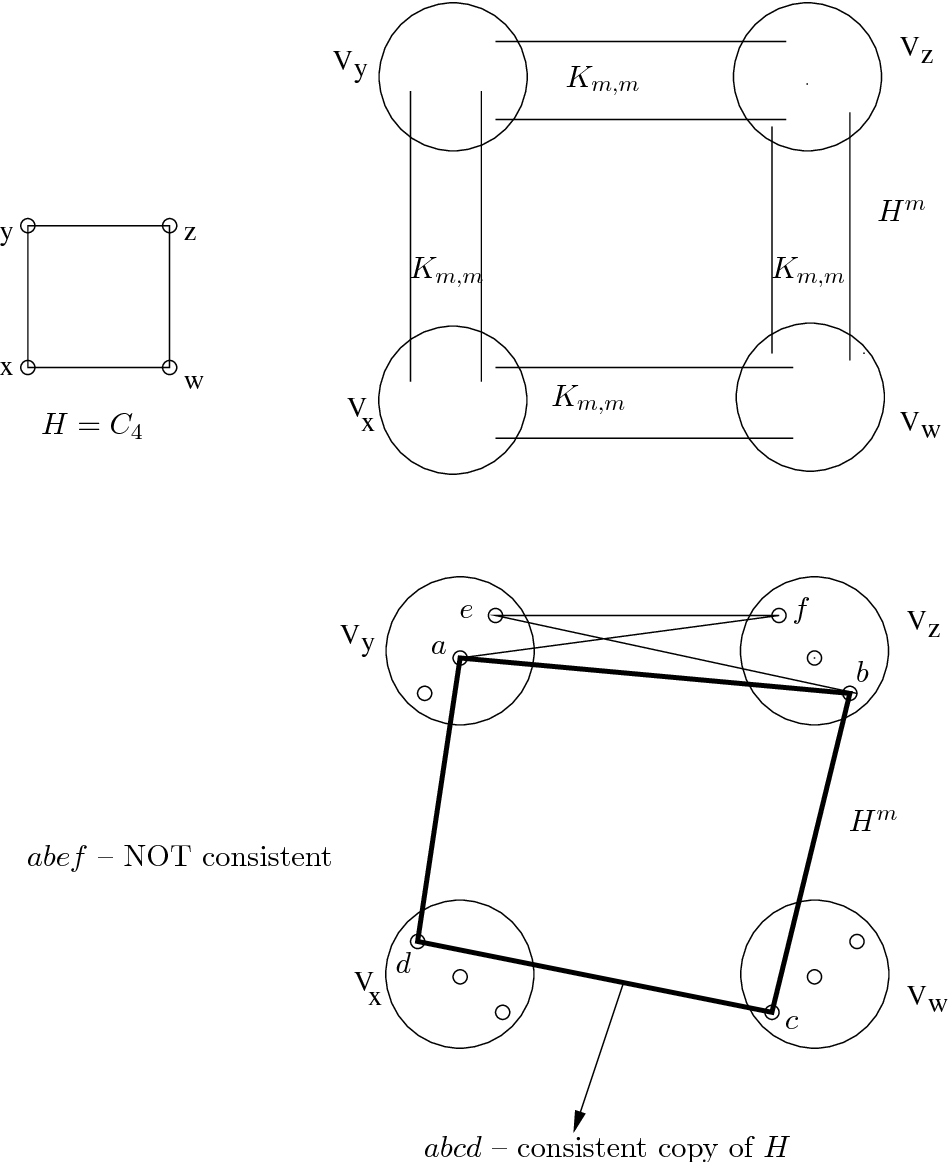Is this relevant?
2003
2003
For a graph G the symbol G->(3,...,3r) means that in every r-colouring of the vertices of G there exists a monochromatic triangle… Expand
•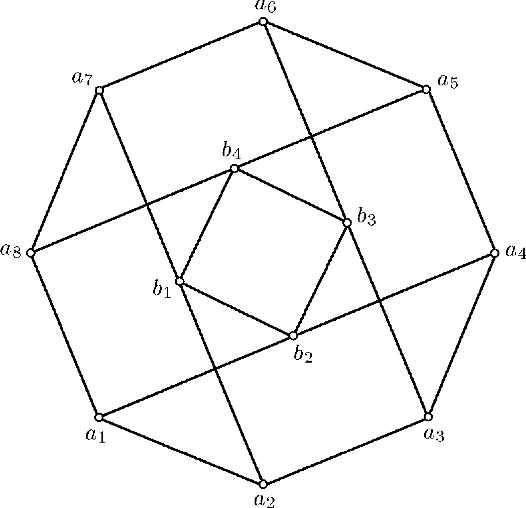Is this relevant?
1996
1996
Let F (n, k) denote the maximum number of two edge colorings of a graph on n vertices that admit no monochromatic Kk, (a complete… Expand
Is this relevant?
1994
1994
• Random Struct. Algorithms
• 1994
• Corpus ID: 26655929
We prove that for every r ⩾ 2 there is C > 0 such that if pCn−1/2 then almost surely every r-coloring of the edges of the… Expand
Is this relevant?